We have derived the velocity addition formula using two Lorentz transformations (in the same direction) and rapidity.We can also compute this in terms of the usualgoing from one frame in which an object is moving to a frame boosted along thedirection. (This should give the same result as above if the particle is moving in the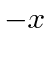direction.)

Let the velocity in the original system be.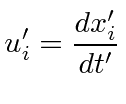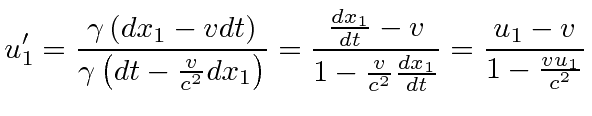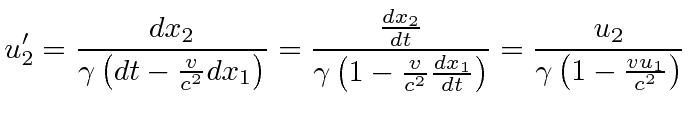This formula gives the same result as above but is more general since it allows us to add velocities that are in different directions.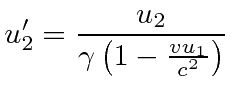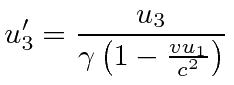Jim Branson 2012-10-21